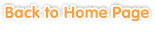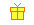Sample questions are shown below. Just click to start!• Chapter 1
Multiplying 3-Digit Numbers with 2-Digit Numbers
••
Using Paper and Pencil• Chapter 2
Dividing Multiple-Digit Numbers with 2-Digit Numbers
•
Mental Math•• Chapter 3• Chapter 4
Arithmetic Operations
•• Chapter 5
Basic Number Properties
••• Chapter 6
Decimals
••
Moving Decimal Point•
Place Value•
Comparing Decimals••••
Estimating and Rounding• Chapter 7
Problem Solving: Adding and Subtracting Decimals• Chapter 8
Large Numbers
•
Place Value and Expanded Form• Chapter 9
Geometry
•••• Chapter 10
Statistics
•